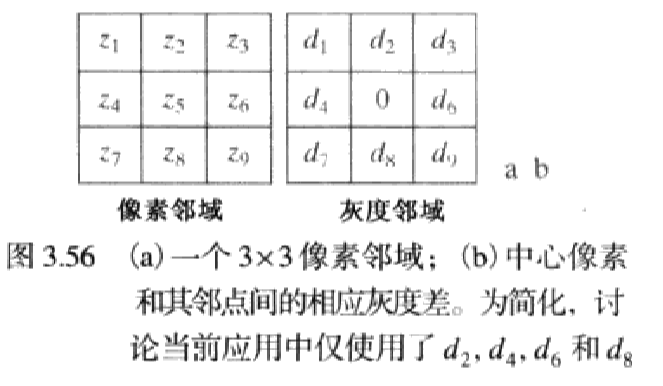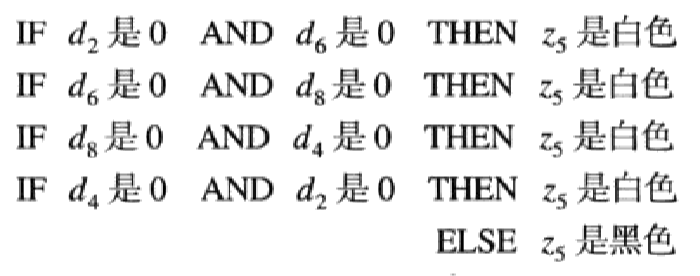2018-12-20 10:58:40 qq_41881554 阅读数 422
• ###### MATLAB图像处理

全面系统的学习MATLAB在图像处理中的应用

20342 人正在学习 去看看 魏伟

# 简要原理

如下图所示，我们将一个像素8个相邻的像素，各减去这个像素的值，即，di=di-z5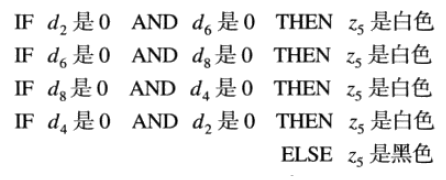其中，“0”集合（ZE）的隶属度函数为

# 示例：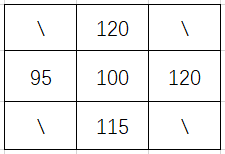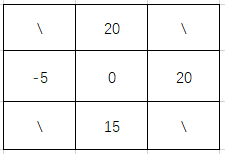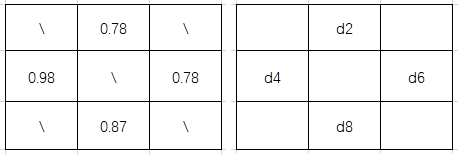wh=max(wh1,wh2,wh3,wh4)=0.87

bl=1-wh=0.13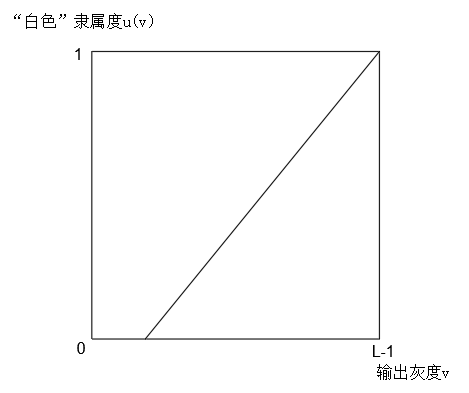Q(1)=min(wh,u(v))，如下图：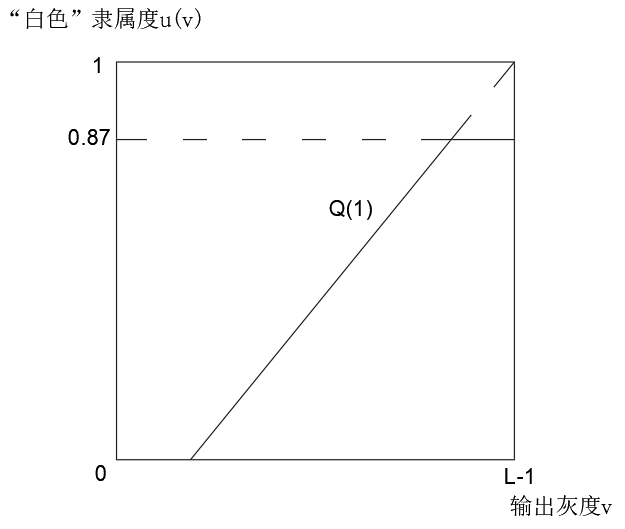Q(2)=min(bl,u(v))，如下图：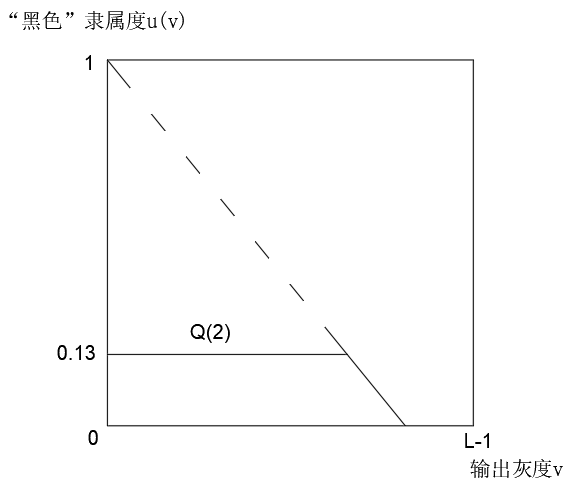Q = max(Q(1)，Q(2))，如下图：

v0=174.405

# 代码：

VS2013+OpenCV3.0代码如下：

/*
***************************************************************************************************************************************
-----------------------------------------------作者：我三食堂不服----------------------------------------------------------------------
***************************************************************************************************************************************
*/

#include <opencv2/opencv.hpp>
#include <math.h>
#include <vector>

using namespace std;
using namespace cv;

/*
//模糊集合的边缘检测
//isUnify - 是否标定（仅在位深度为8时有效），false - 梯度值小于0时置0，大于255时置为255，true - 梯度值拉伸到0 ~ 255内
*/
int FuzzyEdge(const Mat &input, Mat &out, bool isUnify = false);

int main(int argc, char** argv)
{
Mat im_source;				//原图
Mat im_pro1;				//处理后的图片
Mat im_pro2;				//处理后的图片

//载入原图（灰度图方式）

//图片处理
FuzzyEdge(im_source, im_pro1);
FuzzyEdge(im_source, im_pro2, true);

//创建窗口
namedWindow("【原图】");
namedWindow("【处理后1】");
namedWindow("【处理后2】");

//显示图片
imshow("【原图】", im_source);
imshow("【处理后1】", im_pro1);
imshow("【处理后2】", im_pro2);

//保存图片
imwrite("3-59_b.tif", im_pro1);
imwrite("3-59_c.tif", im_pro2);

//等待键盘操作
cvWaitKey(0);

return 0;
}

/*
//模糊集合的边缘检测
//isUnify - 是否标定（仅在位深度为8时有效），false - 梯度值小于0时置0，大于255时置为255，true - 梯度值拉伸到0 ~ 255内
*/
int FuzzyEdge(const Mat &input, Mat &out, bool isUnify)
{
double kernel;					// 领域灰度差\隶属度
double WH, BL;			// 颜色隶属度函数
double wh1, wh2, wh3, wh4, wh, bl;	// 各条件对应白色（黑色）隶属度
double Q, MQ;						// 输出模糊集合的隶属度、隶属度总和
Mat extend;							// 对输入图像进行拓展
Mat out_f;							// 输出图像
uchar* data_extend;					// 拓展的图像像素数据
uchar* data_out;					// 输出图片像素数据
long double data;					// 灰度值
int h{ input.rows };				// 图片高
int w{ input.cols };				// 图片宽
int w2{ w + 2 };					// 拓展图片宽
int h2{ h + 2 };					// 拓展图片高
int u, v, i;						// 循环变量

//参数输入错误
if (h < 3 || w < 3)
return 0;

//拓展图像
copyMakeBorder(input, extend, 1, 1, 1, 1, BORDER_REFLECT_101);
data_extend = extend.data;

//输出图像初始化
out.release();
out.create(h, w, input.type());
out_f.create(h, w, CV_32FC1);
data_out = out.data;

//颜色隶属度函数初始化
for (i = 0; i < 256; i++)
{
if (i < 204)
BL[i] = (204.0 - i) / 204.0;
else
BL[i] = 0;

if (i>51)
WH[i] = (i - 51.0) / 204.0;
else
WH[i] = 0;
}

//进行边缘增强
for (v = 1; v < h2 - 1; v++)
for (u = 1; u < w2 - 1; u++)
{
//计算灰度差(即d2,d4,d6,d8)
kernel = data_extend[(v - 1)*w2 + u] - data_extend[v*w2 + u];
kernel = data_extend[(v)*w2 + u - 1] - data_extend[v*w2 + u];
kernel = data_extend[(v)*w2 + u + 1] - data_extend[v*w2 + u];
kernel = data_extend[(v + 1)*w2 + u] - data_extend[v*w2 + u];

//计算d2,d4,d6,d8四个灰度差属于
//“灰度差为0”集合
//的隶属度
for (i = 0; i < 3; i++)
{
kernel[i] /= 255;
kernel[i] = exp(-40 * kernel[i] * kernel[i]);
}

//计算各像素属于
//“di和dj同时为0”集合（i,j=2、4、6、8）
//的隶属度
wh1 = min(kernel, kernel);
wh2 = min(kernel, kernel);
wh3 = min(kernel, kernel);
wh4 = min(kernel, kernel);

//计算各像素属于
//“d2,d4,d6,d8全为0”集合
//的隶属度
wh = max(max(wh1, wh2), max(wh3, wh4));

//计算各像素属于
//“满足第5个条件”集合
//的隶属度
bl = 1 - wh;

//计算输出灰度(重心)
data = 0;
MQ = 0;
for (i = 0; i < 256; i++)
{
Q = max(min(BL[i], bl), min(WH[i], wh));
data += i*Q;
MQ += Q;
}
data /= MQ;
out_f.at<float>(v - 1, u - 1) = (float)data;
}

//图像格式转换
if (isUnify)
{
double max, min, size, k;
minMaxIdx(out_f, &min, &max);
size = max - min;
k = 255 / size;

for (v = 0; v < h; v++)
for (u = 0; u < w; u++)
{
data_out[v*w + u] = (uchar)(k*(out_f.at<float>(v, u) - min));
}
}
else
{
for (v = 0; v < h; v++)
for (u = 0; u < w; u++)
{
data = out_f.at<float>(v, u);
if (data < 0)
data = 0;
else if (data>255)
data = 255;

data_out[v*w + u] = (uchar)data;
}
}

return 0;
}


# 运行效果：

2019-10-14 09:12:37 weixin_35732969 阅读数 739
• ###### MATLAB图像处理

全面系统的学习MATLAB在图像处理中的应用

20342 人正在学习 去看看 魏伟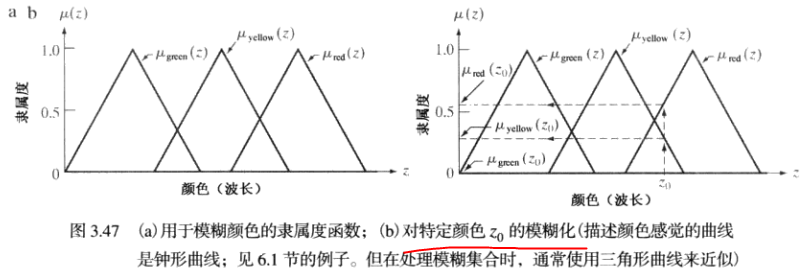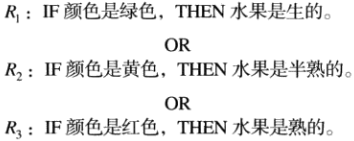IF-THEN规则中，THEN左侧部分称为前提条件），右侧部分称为后果结论）。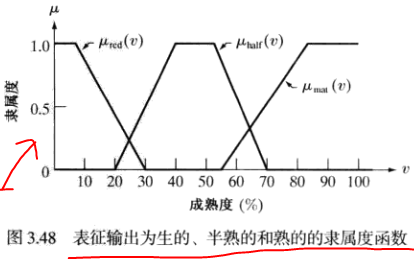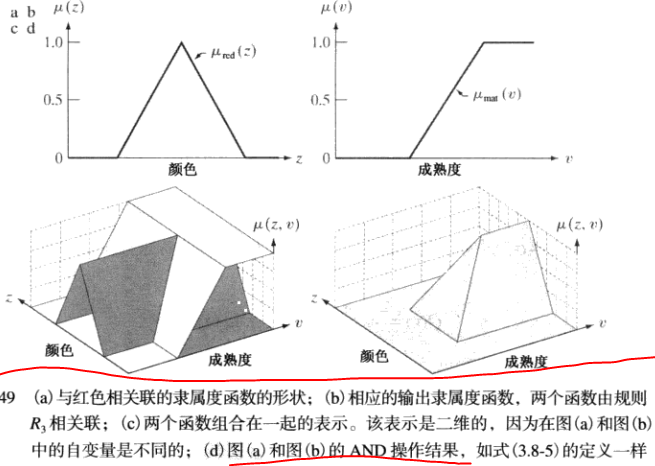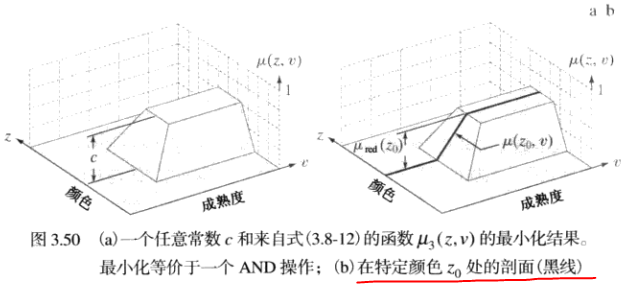OR写成最大操作的形式：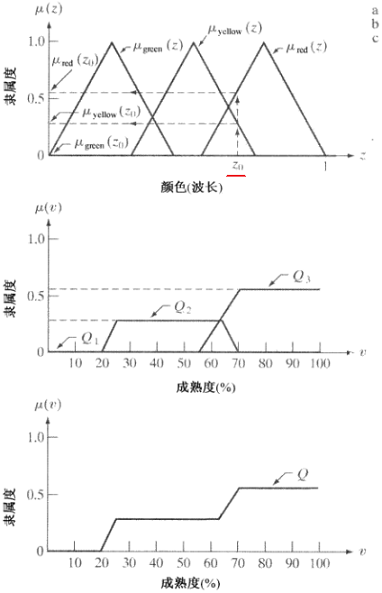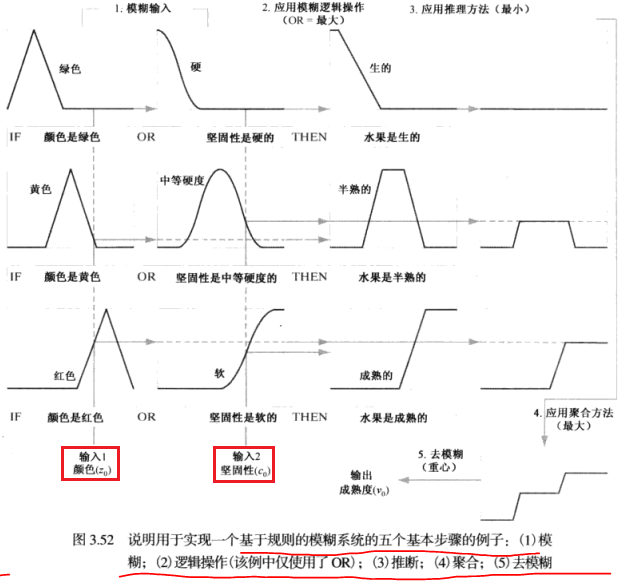THEN规则的条件很难满足时，执行ELSE规则。ELSE规则的强度水平如下：

IF 一个像素是暗的，THEN 使它较暗；

IF 一个像素是灰的，THEN 使它仍是灰的；

IF 一个像素是亮的，THEN 使它较亮。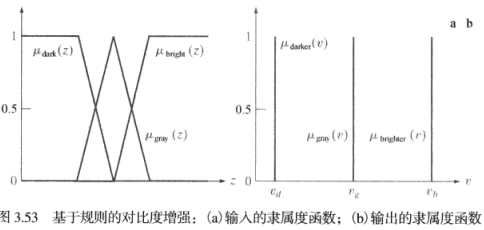2018-02-28 10:32:18 zhengxiaoyang995926 阅读数 313
• ###### MATLAB图像处理

全面系统的学习MATLAB在图像处理中的应用

20342 人正在学习 去看看 魏伟

Ac={(x, y, K-z)|(x, y, z)∈A}；

A= Ac={(x, y, 255-z)|(x, y, z)∈A};

A∪B = {max(a, b)|a∈A, b∈B};

U代表所有的人，令AU的子集，为年轻人的集合。引入隶属度函数对U中每个元素赋值。隶属度函数简单地定义为一个阈值，低于该阈值的为年轻人，高于该阈值的为非年轻人。思考：假设20岁为阈值，20岁为年轻人，20岁零一秒就不是年轻人了？所以需要更多的灵活性，即从年轻到非年轻过渡。使用模糊集合可以声明一个人的年轻度为50％。换句话说，年龄是一个不精确的概念，而模糊逻辑提供处理这种概念的工具。

2019-10-14 09:14:08 weixin_35732969 阅读数 802
• ###### MATLAB图像处理

全面系统的学习MATLAB在图像处理中的应用

20342 人正在学习 去看看 魏伟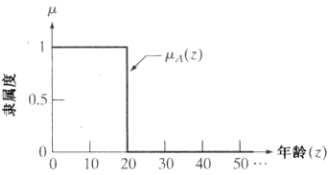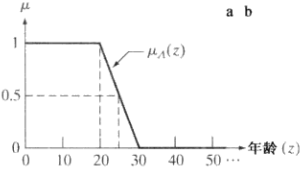A = {(1,1),(2,1),(3,1),...,(20,1),(21,0.9),(22,0.8),...,(25,0.5),(26,0.4),...,(29,0.1)}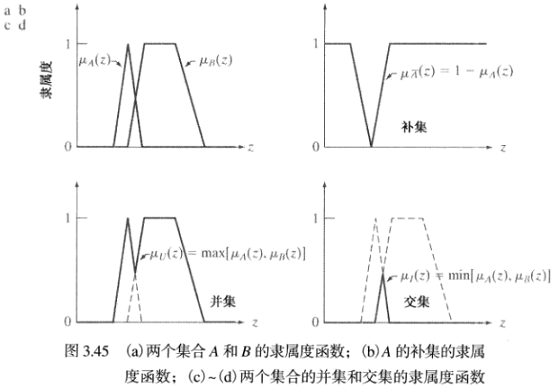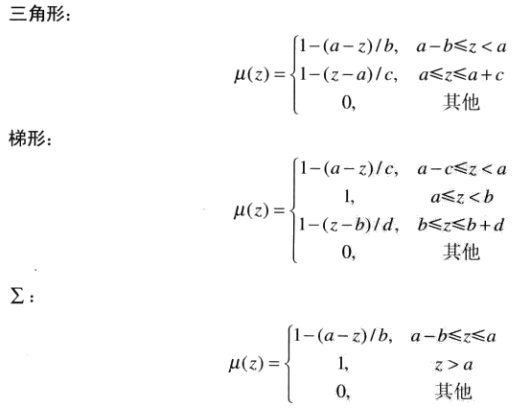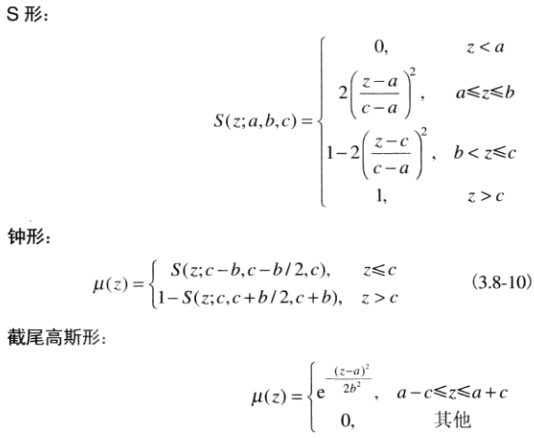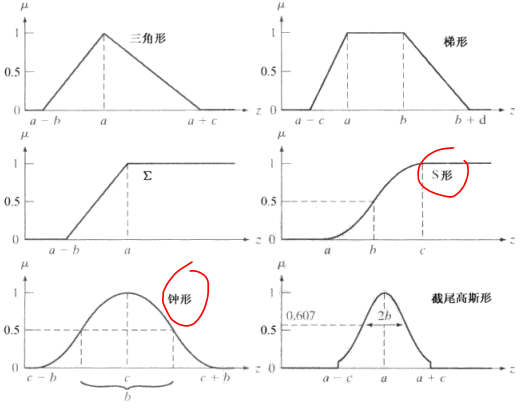2019-05-18 10:30:28 yishi_c 阅读数 162
• ###### MATLAB图像处理

全面系统的学习MATLAB在图像处理中的应用

20342 人正在学习 去看看 魏伟

### 使用模糊技术进行灰度变换和空间滤波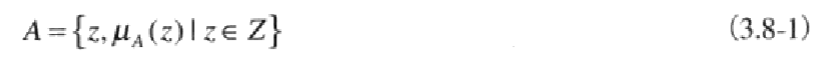### 1.模糊集合论原理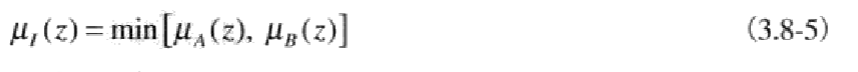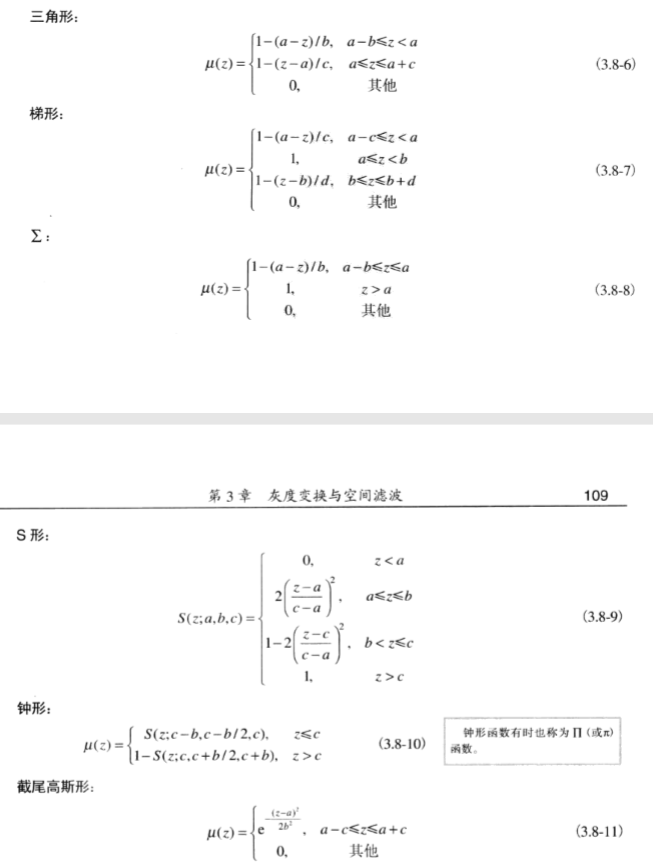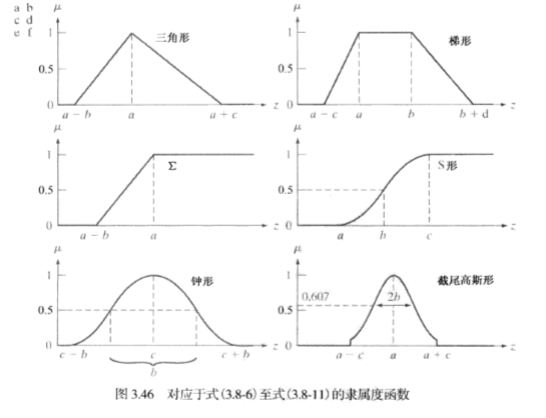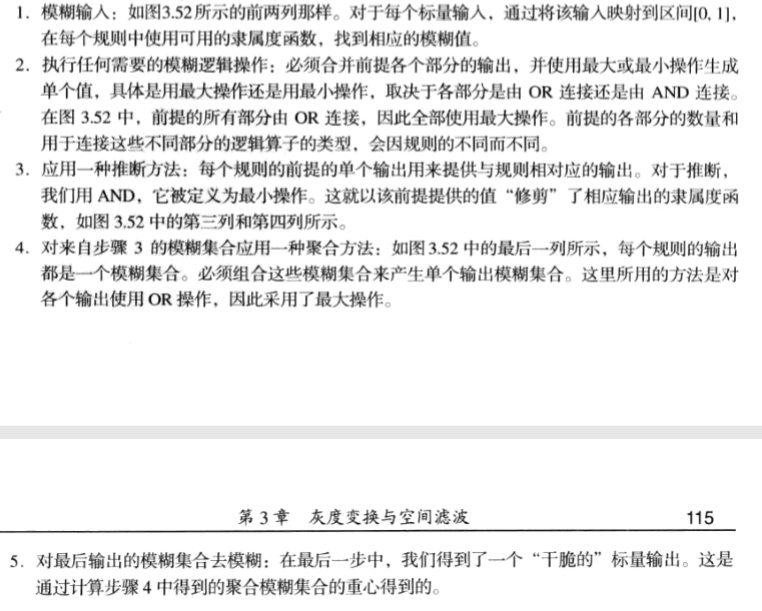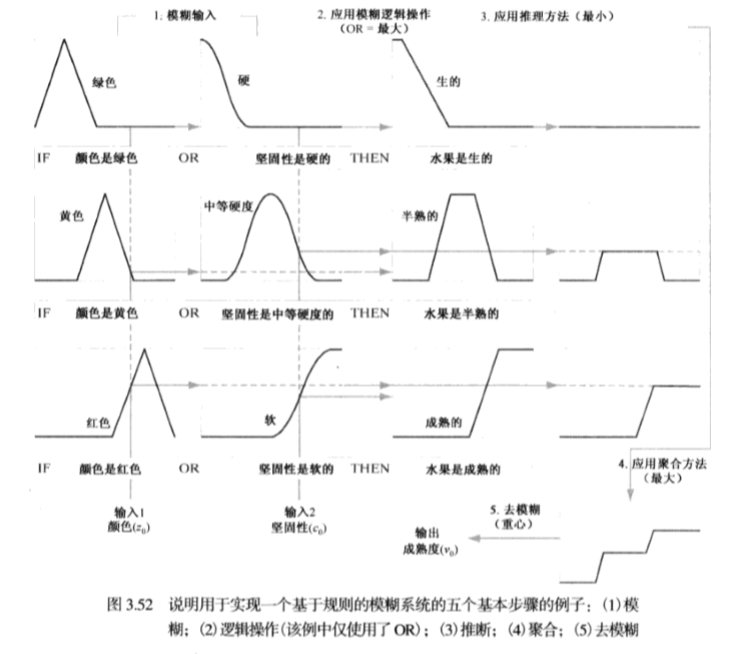### 2.使用模糊集合进行灰度变换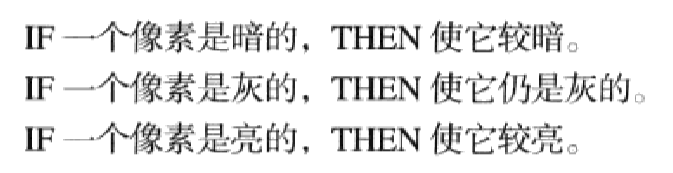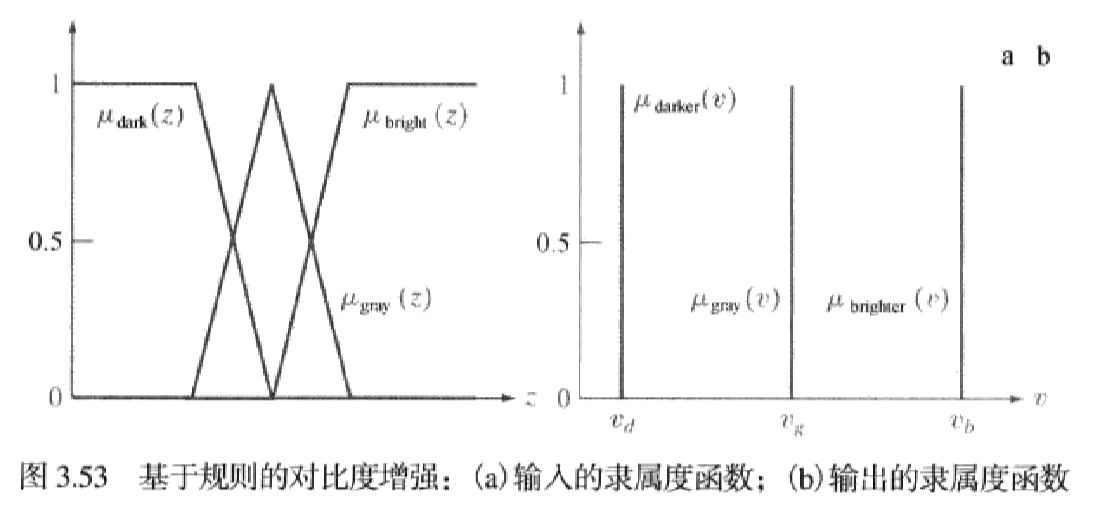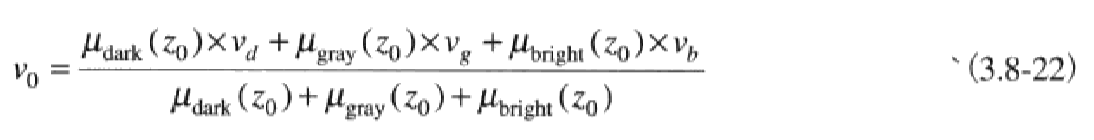### 3.使用模糊集合进行空间滤波